# Hedging Overview

Hedging in Fairways Debt presents the relationship of the actual debt and the risk hedging transactions.

1. Navigate to Applications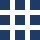> Debt & Derivatives > Hedging.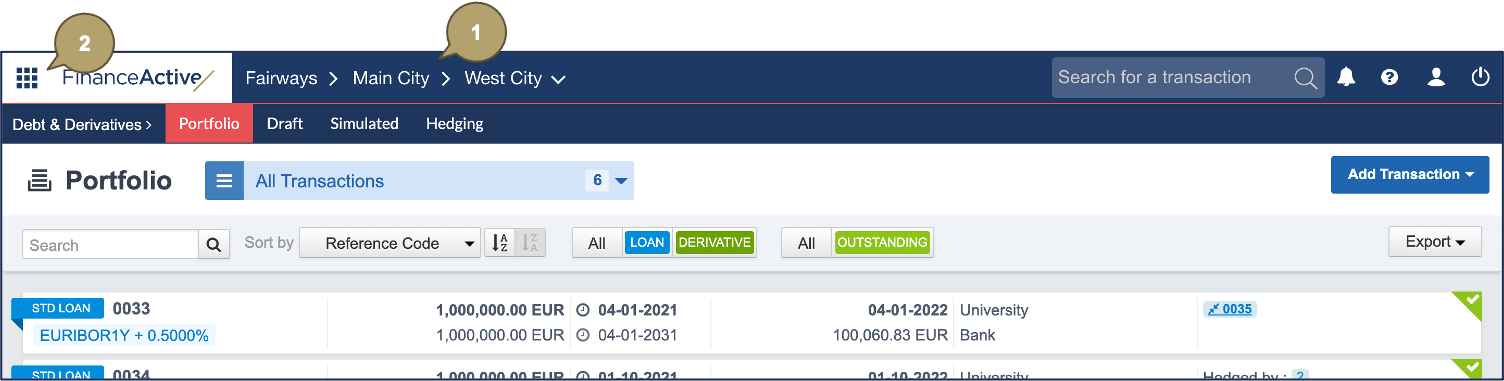The hedging overview displays.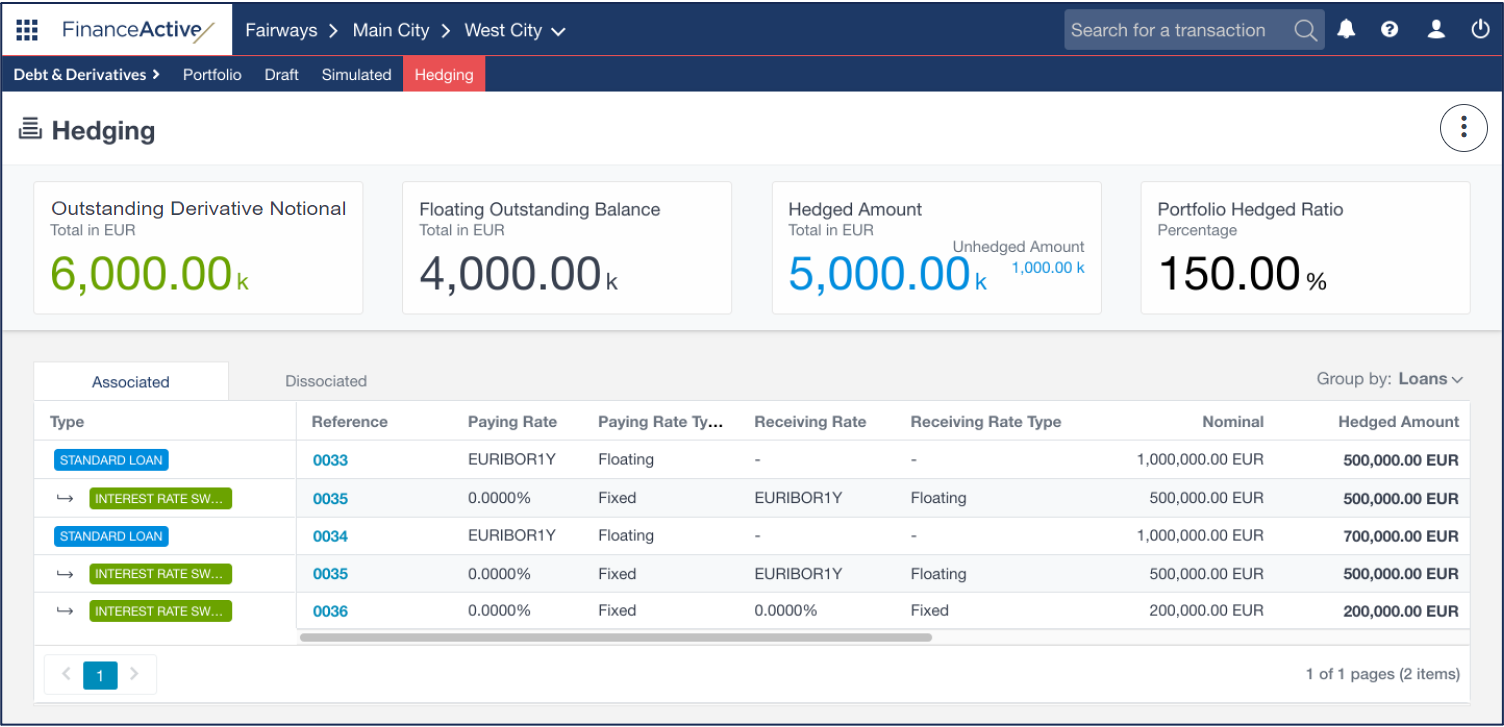Indicator

Description

Outstanding Derivative Notional

Total notional amount of all the outstanding derivatives in the actual portfolio.

Floating Outstanding Balance

Total balance amount of all the outstanding floating-rate transactions in the actual portfolio.

Hedged Amount

Total notional amount of the derivatives associated with at least one hedged transaction.

In this example, the hedged amount is EUR 5 000 000.

We add up the notionals of all derivatives displayed in the Associated list:

2 000 000 + 3 000 000

= 5 000 000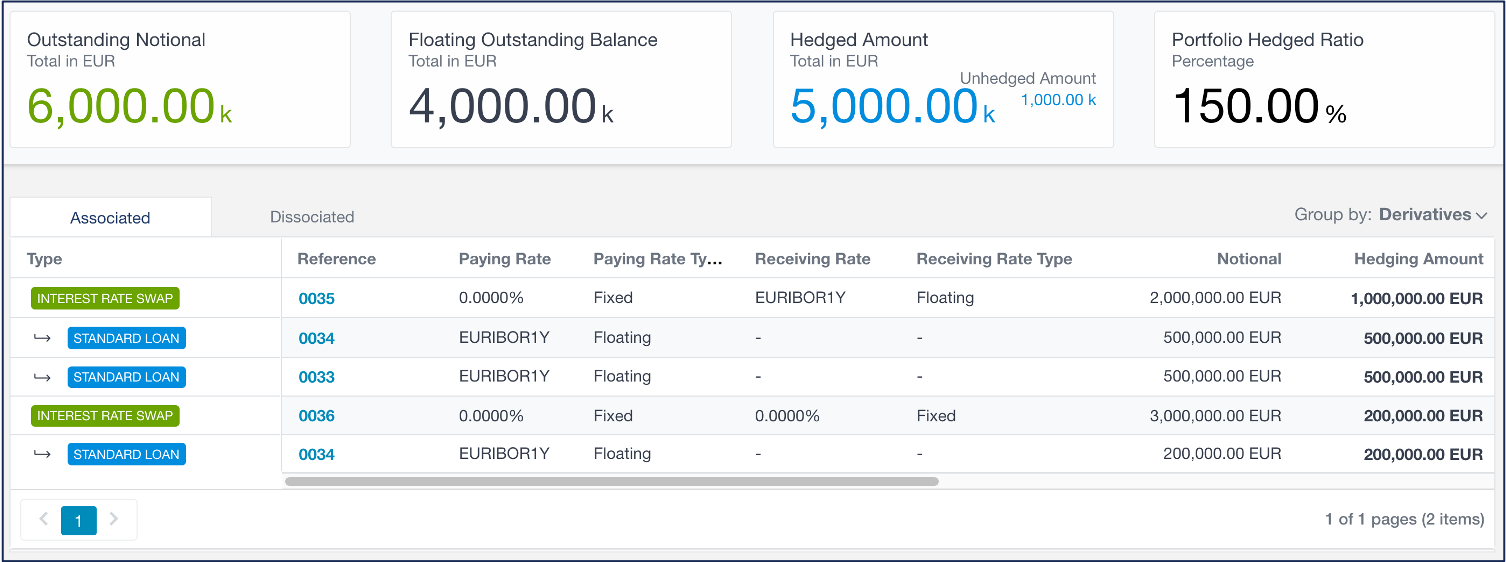Unhedged Amount

Total notional amount of the derivatives associated with no hedged transaction:

Outstanding Derivative Notional - Hedged Amount

In this example, the unhedged amount is EUR 1 000 000.

Outstanding Derivative Notional - Hedged Amount

= 6 000 000 - 5 000 000

= 1 000 000Portfolio Hedged Ratio

Ratio between hedgeable amount (notional) and amount to hedge (balance):

Outstanding Derivative Notional / Floating Outstanding Balance * 100

In this example, the portfolio hedged ratio is 150%.

Outstanding Derivative Notional / Floating Outstanding Balance * 100

= 6 000 000 / 4 000 000 * 100

= 150%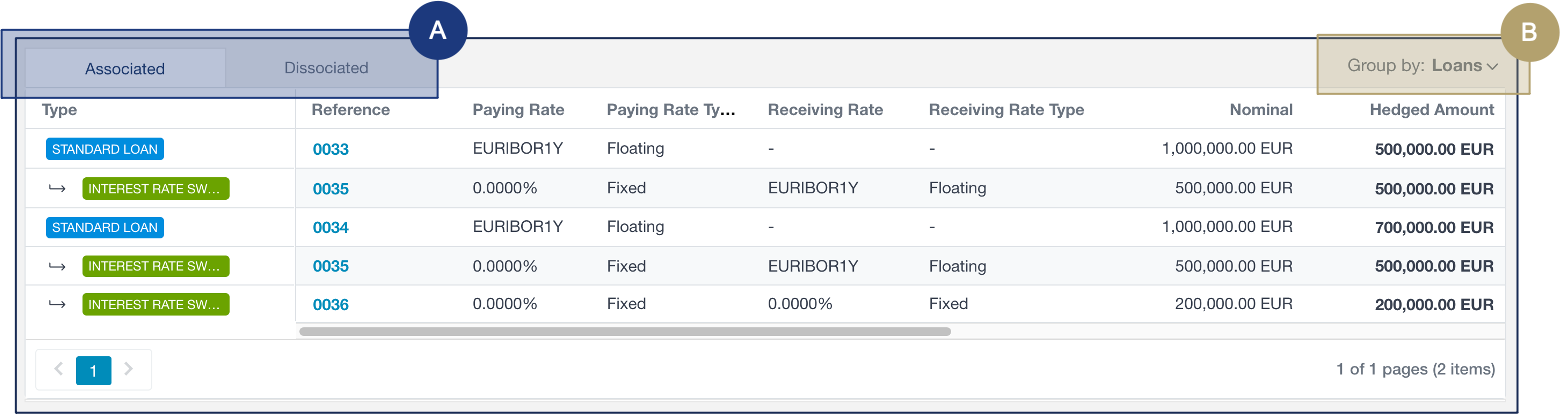#

Description

Options

A

Display:

• Associated: Transactions currently hedged, i.e. transactions associated with derivatives.
• Dissociated:
• Transactions not currently associated with any derivatives.
• Derivatives not currently associated with any transactions.

B

Group transactions to sort them in the list.

• Loans
• Derivatives
• Derivative Currencies
• Derivative Counterparties